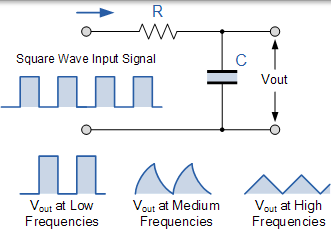# 无源低通滤波器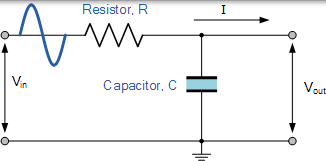• 低通滤波器 - 低通滤波器只允许低频信号从 0Hz 到其截止频率 ƒc 通过，同时阻止那些更高的频率。
• 高通滤波器 - 高通滤波器仅允许高频信号从其截止频率 ƒc 开始到无限高频通过，同时阻止任何更低的信号。
• 带通滤波器 - 带通滤波器允许在两点之间设置的特定频带内的信号通过，同时阻挡该频带两侧的较低和较高频率。

### 理想的滤波器响应曲线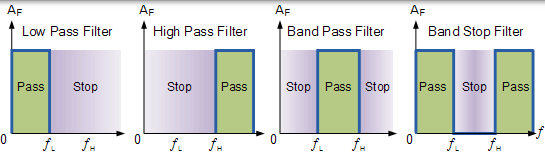## 低通滤波器

### RC 低通滤波器电路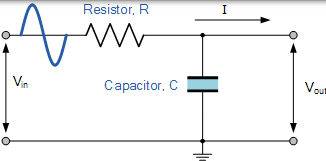$$\mathrm { V } _ { \mathrm { out } } = \mathrm { V } _ { \mathrm { in } } \times \frac { \mathrm { R } _ { 2 } } { \mathrm { R } _ { 1 } + \mathrm { R } _ { 2 } }$$

$$\mathrm { X } _ { \mathrm { C } } = \frac { 1 } { 2 \pi \mathrm { f } \mathrm { C } } \quad \mathrm { \Omega }$$

$$Z = \sqrt { R ^ { 2 } + X _ { C } ^ { 2 } }$$

### RC 电势分压方程

$$\mathrm { V } _ { \mathrm { out } } = \mathrm { V } _ { \mathrm { in } } \times \frac { \mathrm { X } _ { \mathrm { C } } } { \sqrt { \mathrm { R } ^ { 2 } + \mathrm { X } _ { \mathrm { C } } ^ { 2 } } } = \mathrm { V } _ { \mathrm { in } } \frac { \mathrm { X } _ { \mathrm { C } } } { \mathrm { Z } }$$

## 低通滤波器示例 No1

### 电压输出频率为 100Hz

$$\mathrm { Xc } = \frac { 1 } { 2 \pi \mathrm { f } \mathrm { C } } = \frac { 1 } { 2 \pi \times 100 \times 47 \times 10 ^ { - 9 } } = 33,863 \Omega$$

$$\mathrm { V } _ { \mathrm { ouT } } = \mathrm { V } _ { \mathrm { IN } } \times \frac { \mathrm { Xc } } { \sqrt { \mathrm { R } ^ { 2 } + \mathrm { X } _ { \mathrm { C } } ^ { 2 } } } = 10 \times \frac { 33863 } { \sqrt { 4700 ^ { 2 } + 33863 ^ { 2 } } } = 9.9 \mathrm { v }$$

### 电压输出频率为 10,000Hz(10kHz)

$$\mathrm { X } _ { \mathrm { c } } = \frac { 1 } { 2 \pi \mathrm { f } \mathrm { C } } = \frac { 1 } { 2 \pi \times 10,000 \times 47 \times 10 ^ { - 9 } } = 338.6 \Omega$$

$$\mathrm { V } _ { \mathrm { ouT } } = \mathrm { V } _ { \mathrm { II } } \times \frac { \mathrm { X } _ { \mathrm { C } } } { \sqrt { \mathrm { R } ^ { 2 } + \mathrm { X } _ { \mathrm { C } } ^ { 2 } } } = 10 \times \frac { 338.6 } { \sqrt { 4700 ^ { 2 } + 338.6 ^ { 2 } } } = 0.718 \mathrm { v }$$

## 频率响应

### 一阶低通滤波器的频率响应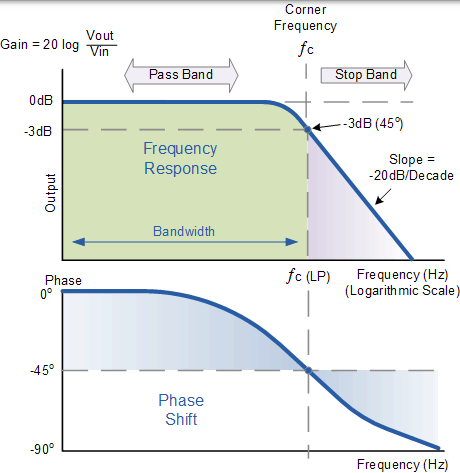### 截止频率和相移

$${ f \mathrm { c } = \frac { 1 } { 2 \pi \mathrm { RC } } = \frac { 1 } { 2 \pi \times 4700 \times 47 \times 10 ^ { - 9 } } = 720 \mathrm { Hz } }$$

$${ \text { Phase Shift } \varphi = - \arctan ( 2 \pi f \mathrm { RC } ) }$$

## 二阶低通滤波器

### 二阶低通滤波器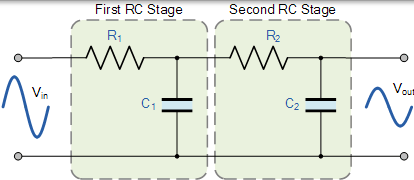### ƒc 的无源低通滤波器增益

$$\left( \frac { 1 } { \sqrt { 2 } } \right) ^ { n }$$

### 二阶滤波器转角频率

$$f _ { \mathrm { C } } = \frac { 1 } { 2 \pi \sqrt { \mathrm { R } _ { 1 } \mathrm { C } _ { 1 } \mathrm { R } _ { 2 } \mathrm { C } _ { 2 } } } \mathrm { Hz }$$

### 二阶低通滤波器 -3dB 频率

$$f _ { ( - 3 d B ) } = f _ { c } \sqrt { 2 ^ { \frac { 1 } { n } } - 1 }$$

### 二阶低通滤波器的频率响应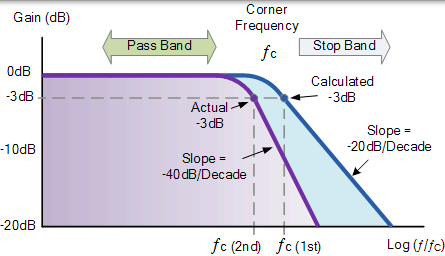## 低通滤波器摘要

$$\text {Gain in } d B = 20 \log \frac { V o u t } { V i n }$$

## 时间常数

$$\tau = R C = \frac { 1 } { 2 \pi f c }$$

$$f _ { C } = \frac { 1 } { 2 \pi R C } \text { or } \frac { 1 } { 2 \pi \tau }$$

## RC 积分器

### RC 积分电路